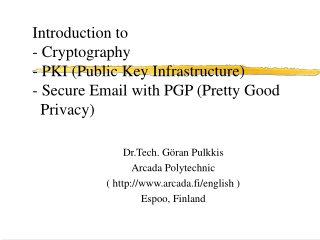DownloadDownload PresentationDownload Presentation- - - - - - - - - - - - - - - - - - - - - - - - - - - E N D - - - - - - - - - - - - - - - - - - - - - - - - - - -
##### Presentation Transcript

1. Introduction to - Cryptography - PKI (Public Key Infrastructure)- Secure Email with PGP (Pretty Good Privacy) Dr.Tech. Göran Pulkkis Arcada Polytechnic ( http://www.arcada.fi/english ) Espoo, Finland

2. Cryptographic algorithms

3. Secret Key Cryptography

4. Public Key Cryptography

5. Mathematical basis of cryptography • information theory • theory of computational complexity • integer algebra (modulo arithmetics, factoring, random number generation, prime number generation and discrete logarithms)

6. Information theory • Confusion obscures the relationships between the plaintext and the encrypted ciphertext. The simplest confusion technique is substitution of binary patterns • Diffusion speads local information content of plaintext over the entire encrypted ciphertext. The simplest confusion technique is permutation of bit patterns

7. Quality Measures of Cryptosystems

8. Theory of Computational Complexity • the computation time and memory space requirements of encryption and decryption operations • the computation time requirements for breaking a cryptosystem

9. Ideal Cryptographic Algorithms • encryption and decryption operations have low complexity (=short execution times) • encryption can be broken only by guessing the correct key or trying out all possible keys, if the correct key is unknown (=exponential complexity - cracking time proportional to 2n where n is the number of all possible keys)

10. Strong Encryption

11. A useful cryptographic algorithm • it has been mathematically proved to be ideal or • it has been for years been and is still being thoroughly studied in public research by many acknowledged cryptoanalysts all over the world and no easy cracking method (= estimated deterministic complexity lower than superpolynomial) is known in public

12. Conclusions for Applied Cryptography

13. Secret Key Algorithms in Use • Triple DES (Data Encryption Standard) with key length 112 or 168 bit • IDEA (International Data Encryption Standard) with key length 128 bits

14. Public Key Algorithms in Use • RSA (Rivest-Shamir-Adleman) with key length ³ 1024 bits • ECC (Elliptic Curve Cryptography) based algorithms with key length ³ 160 bits

15. Security of public key cryptosystems • irreproducible generation of large (>150 digits) random primes • the high computational complexity of the problem to find the factors of a large (>300 digits) integer • the high computational complexity of the problem to find the discrete logarithm in modulo arithmetics when the modulus is a large (>300 digits) integer

16. Modulo Arithmetics

17. Generation of large random primes • An approach to secure random number generation is based on recording irreproducible physical randomness, like mouse movement traces, keystroke time intervals, physical noise, etc. • proved: number of primes  n/ln(n) when n. Thus there exists about 10154/(154*ln(10))  3*10151 positive primes which can be coded by 512 bits (“only” 1077 atoms in Universe). One of 300 numbers is on the average a prime! • large integers can efficiently be checked for primality in a PC (some milliseconds). Generate a large random odd integer, then step +2 and check. On average about 300 steps are needed until a prime is found

18. Basic Cryptographic Protocols • Shared Secret Key Generation (Diffie-Hellman Key Exchange) • Digital Signatures - generation and checking • Authentication Protocols (example: Kerberos, implemented in Win2000 logon)

19. Diffie-Hellman (D-H) Key Exchange • Public key algorithms much slower than secret key algorithms (RSA ~1000 times slower than IDEA) • Protected info exchange using a shared secret key • D-H: A and B can agree on a shared secret key by exchanging only unencrypted integer values • Mathematics of D-H: K = (aXA mod p)XB mod p = (aXB mod p)XA mod p, when a (=a generator for modulo base p), p (=a prime), aXA mod p and aXB mod p are exchanded, XA is secret of A, XB is secret of B and K is the shared secret key

20. Digital signature generation • A message/document is combined with an encrypted (private key of signer) hash (a message digest) message message hash algorithm encrypted hash hash encryption Signed message Private key of signer

21. Checking a digital signature • The unencrypted hash of a signed message is compared with the hash of the original message message hash algorithm hash OK if match encrypted hash decryption hash Signed message Public key of signer

22. Hash algorithms • Reduce variable-length messages/documents to fixed-length (usually 128 or 160 bit) hashes • Original info not possible to deduce from a hash • Probability that 2 different messages/documents produce same hash ~ 0 (2160 hash patterns more than atoms in a 6-dimensional Universe, where each atom is replaced by a new Universe) • hash = message digest or fingerprint • Common hash algorithms: MD5 (128 bit), SHA-1 (160 bit), RIPEMD-160 (160 bit)